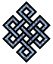#fail2ban bad ip database: ip 194.105.205.42

### | ip database | live view | stats | report | help | api key:

 ip: 194.105.205.42 hostname: 194.105.205.42 country:[RU] Russian Federation first reported: 11.09.2017 02:03.31 GMT+0200 last reported: 23.07.2019 11:09.49 GMT+0200 time period: 680d 09h 06m 18s total reports: 6 reported by: 3 host(s) filter(s): ssh (6) tor exit node no badips.com db Lookup## port scan of '194.105.205.42':

[-hide]
```# Nmap 6.40 scan initiated Mon Sep 11 02:04:02 2017 as: /usr/bin/nmap -sU -sS -O 194.105.205.42
Nmap scan report for 194.105.205.42
Host is up (0.039s latency).
Not shown: 1000 open|filtered ports, 994 filtered ports
PORT     STATE  SERVICE
22/tcp   open   ssh
113/tcp  closed ident
1723/tcp open   pptp
8000/tcp closed http-alt
8443/tcp open   https-alt
8888/tcp open   sun-answerbook
No exact OS matches for host (If you know what OS is running on it, see http://nmap.org/submit/ ).
TCP/IP fingerprint:
OS:SCAN(V=6.40%E=4%D=9/11%OT=22%CT=113%CU=%PV=N%G=Y%TM=59B5D328%P=x86_64-pc
OS:-linux-gnu)SEQ(SP=103%GCD=1%ISR=108%TI=Z%TS=7)OPS(O1=M5B4ST11NW7%O2=M5B4
OS:ST11NW7%O3=M5B4NNT11NW7%O4=M5B4ST11NW7%O5=M5B4ST11NW7%O6=M5B4ST11)WIN(W1
OS:=7120%W2=7120%W3=7120%W4=7120%W5=7120%W6=7120)ECN(R=Y%DF=Y%TG=40%W=7210%
OS:O=M5B4NNSNW7%CC=Y%Q=)ECN(R=N)T1(R=Y%DF=Y%TG=40%S=O%A=S+%F=AS%RD=0%Q=)T1(
OS:R=N)T2(R=N)T3(R=N)T4(R=N)T5(R=Y%DF=N%TG=40%W=0%S=Z%A=S+%F=AR%O=%RD=0%Q=)
OS:T6(R=N)T7(R=N)U1(R=N)IE(R=Y%DFI=N%TG=FF%CD=S)

OS detection performed. Please report any incorrect results at http://nmap.org/submit/ .
# Nmap done at Mon Sep 11 02:04:56 2017 -- 1 IP address (1 host up) scanned in 55.09 seconds
```
```Σ = 165 | Δt = 0.0051169395446777s
```